## Identify Linear Models Using the Command Line

### Introduction

#### Objectives

Estimate and validate linear models from multiple-input/single-output (MISO) data to find the one that best describes the system dynamics.

After completing this tutorial, you will be able to accomplish the following tasks using the command line:

• Create data objects to represent data.

• Plot the data.

• Process data by removing offsets from the input and output signals.

• Estimate and validate linear models from the data.

• Simulate and predict model output.

Note

This tutorial uses time-domain data to demonstrate how you can estimate linear models. The same workflow applies to fitting frequency-domain data.

#### Data Description

This tutorial uses the data file `co2data.mat`, which contains two experiments of two-input and single-output (MISO) time-domain data from a steady-state that the operator perturbed from equilibrium values.

In the first experiment, the operator introduced a pulse wave to both inputs. In the second experiment, the operator introduced a pulse wave to the first input and a step signal to the second input.### Preparing Data

```load co2data ```

This command loads the following five variables into the MATLAB Workspace:

• `Input_exp1` and `Output_exp1` are the input and output data from the first experiment, respectively.

• `Input_exp2` and `Output_exp2` are the input and output data from the second experiment, respectively.

• `Time` is the time vector from 0 to 1000 minutes, increasing in equal increments of `0.5` min.

For both experiments, the input data consists of two columns of values. The first column of values is the rate of chemical consumption (in kilograms per minute), and the second column of values is the current (in amperes). The output data is a single column of the rate of carbon-dioxide production (in milligrams per minute).

#### Plotting the Input/Output Data

Plot the input and output data from both experiments.

```plot(Time,Input_exp1,Time,Output_exp1) legend('Input 1','Input 2','Output 1') figure plot(Time,Input_exp2,Time,Output_exp2) legend('Input 1','Input 2','Output 1') ```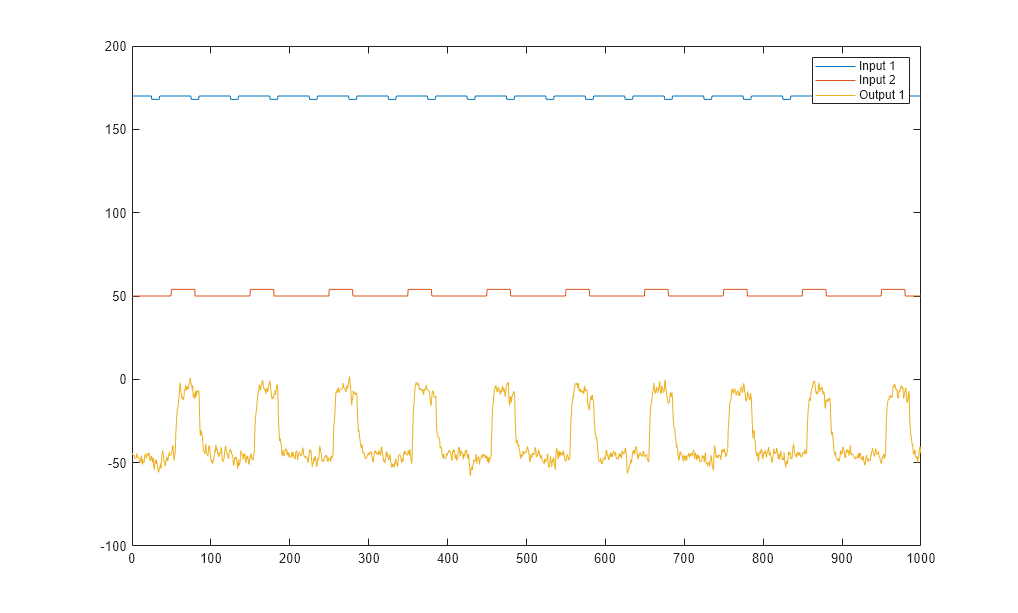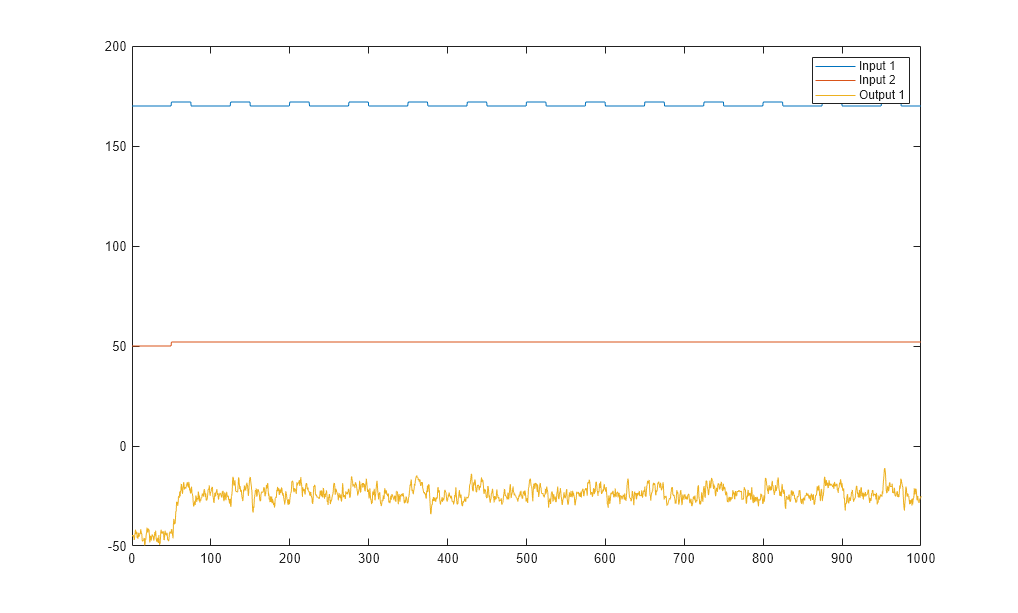The first plot shows the first experiment, where the operator applies a pulse wave to each input to perturb it from its steady-state equilibrium.

The second plot shows the second experiment, where the operator applies a pulse wave to the first input and a step signal to the second input.

#### Removing Equilibrium Values from the Data

Plotting the data, as described in Plotting the Input/Output Data, shows that the inputs and the outputs have nonzero equilibrium values. In this portion of the tutorial, you subtract equilibrium values from the data.

The reason you subtract the mean values from each signal is because, typically, you build linear models that describe the responses for deviations from a physical equilibrium. With steady-state data, it is reasonable to assume that the mean levels of the signals correspond to such an equilibrium. Thus, you can seek models around zero without modeling the absolute equilibrium levels in physical units.

Zoom in on the plots to see that the earliest moment when the operator applies a disturbance to the inputs occurs after 25 minutes of steady-state conditions (or after the first 50 samples). Thus, the average value of the first 50 samples represents the equilibrium conditions.

Use the following commands to remove the equilibrium values from inputs and outputs in both experiments:

```Input_exp1 = Input_exp1-... ones(size(Input_exp1,1),1)*mean(Input_exp1(1:50,:)); Output_exp1 = Output_exp1-... mean(Output_exp1(1:50,:)); Input_exp2 = Input_exp2-... ones(size(Input_exp2,1),1)*mean(Input_exp2(1:50,:)); Output_exp2 = Output_exp2-... mean(Output_exp2(1:50,:)); ```

#### Using Objects to Represent Data for System Identification

The System Identification Toolbox™ data objects, `iddata` and `idfrd`, encapsulate data values and data properties into a single entity. You can use the System Identification Toolbox commands to conveniently manipulate these data objects as single entities.

In this portion of the tutorial, you create two `iddata` objects, one for each of the two experiments. You use the data from Experiment 1 for model estimation, and the data from Experiment 2 for model validation. You work with two independent data sets because you use one data set for model estimation and the other for model validation.

Note

When two independent data sets are not available, you can split one data set into two parts, assuming that each part contains enough information to adequately represent the system dynamics.

#### Creating iddata Objects

Use these commands to create two data objects, `ze` and `zv` :

```Ts = 0.5; % Sample time is 0.5 min ze = iddata(Output_exp1,Input_exp1,Ts); zv = iddata(Output_exp2,Input_exp2,Ts); ```

`ze` contains data from Experiment 1 and `zv` contains data from Experiment 2. `Ts` is the sample time.

The `iddata` constructor requires three arguments for time-domain data and has the following syntax:

`data_obj = iddata(output,input,sampling_interval);`

To view the properties of an `iddata` object, use the `get` command. For example, type this command to get the properties of the estimation data:

```get(ze) ```
```ans = struct with fields: Domain: 'Time' Name: '' OutputData: [2001x1 double] y: 'Same as OutputData' OutputName: {'y1'} OutputUnit: {''} InputData: [2001x2 double] u: 'Same as InputData' InputName: {2x1 cell} InputUnit: {2x1 cell} Period: [2x1 double] InterSample: {2x1 cell} Ts: 0.5000 Tstart: 0.5000 SamplingInstants: [2001x1 double] TimeUnit: 'seconds' ExperimentName: 'Exp1' Notes: {} UserData: [] ```

To learn more about the properties of this data object, see the `iddata` reference page.

To modify data properties, you can use dot notation or the `set` command. For example, to assign channel names and units that label plot axes, type the following syntax in the MATLAB Command Window:

```% Set time units to minutes ze.TimeUnit = 'min'; % Set names of input channels ze.InputName = {'ConsumptionRate','Current'}; % Set units for input variables ze.InputUnit = {'kg/min','A'}; % Set name of output channel ze.OutputName = 'Production'; % Set unit of output channel ze.OutputUnit = 'mg/min'; % Set validation data properties zv.TimeUnit = 'min'; zv.InputName = {'ConsumptionRate','Current'}; zv.InputUnit = {'kg/min','A'}; zv.OutputName = 'Production'; zv.OutputUnit = 'mg/min'; ```

You can verify that the `InputName` property of `ze` is changed, or "index" into this property:

```ze.inputname; ```

Tip

Property names, such as `InputUnit`, are not case sensitive. You can also abbreviate property names that start with `Input` or `Output` by substituting `u` for `Input` and `y` for `Output` in the property name. For example, `OutputUnit` is equivalent to `yunit`.

#### Plotting the Data in a Data Object

You can plot `iddata` objects using the `plot` command.

Plot the estimation data.

```plot(ze) ```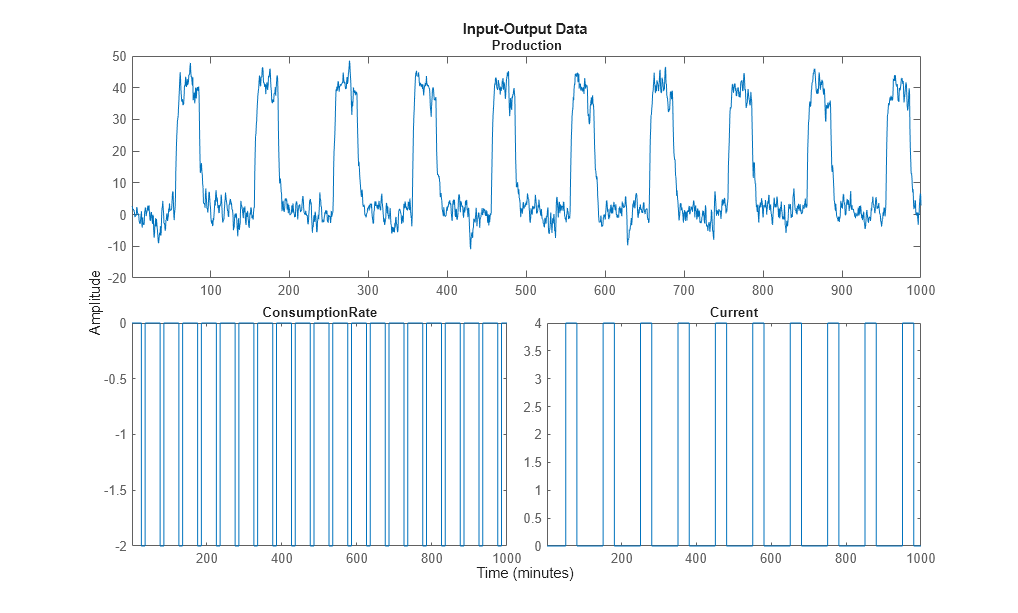The bottom axes show inputs `ConsumptionRate` and `Current`, and the top axes show the output `ProductionRate`.

Plot the validation data in a new figure window.

```figure % Open a new MATLAB Figure window plot(zv) % Plot the validation data ```Zoom in on the plots to see that the experiment process amplifies the first input ( `ConsumptionRate` ) by a factor of 2, and amplifies the second input (`Current` ) by a factor of 10.

#### Selecting a Subset of the Data

Before you begin, create a new data set that contains only the first 1000 samples of the original estimation and validation data sets to speed up the calculations.

```Ze1 = ze(1:1000); Zv1 = zv(1:1000); ```

For more information about indexing into `iddata` objects, see the corresponding reference page.

### Estimating Impulse Response Models

#### Why Estimate Step- and Frequency-Response Models?

Frequency-response and step-response are nonparametric models that can help you understand the dynamic characteristics of your system. These models are not represented by a compact mathematical formula with adjustable parameters. Instead, they consist of data tables.

In this portion of the tutorial, you estimate these models using the data set `ze`. You must have already created `ze`, as described in Creating iddata Objects.

The response plots from these models show the following characteristics of the system:

• The response from the first input to the output might be a second-order function.

• The response from the second input to the output might be a first-order or an overdamped function.

#### Estimating the Frequency Response

The System Identification Toolbox product provides three functions for estimating the frequency response:

• `etfe` computes the empirical transfer function using Fourier analysis.

• `spa` estimates the transfer function using spectral analysis for a fixed frequency resolution.

• `spafdr` lets you specify a variable frequency resolution for estimating the frequency response.

Use `spa` to estimate the frequency response.

```Ge = spa(ze); ```

Plot the frequency response as a Bode plot.

```bode(Ge) ```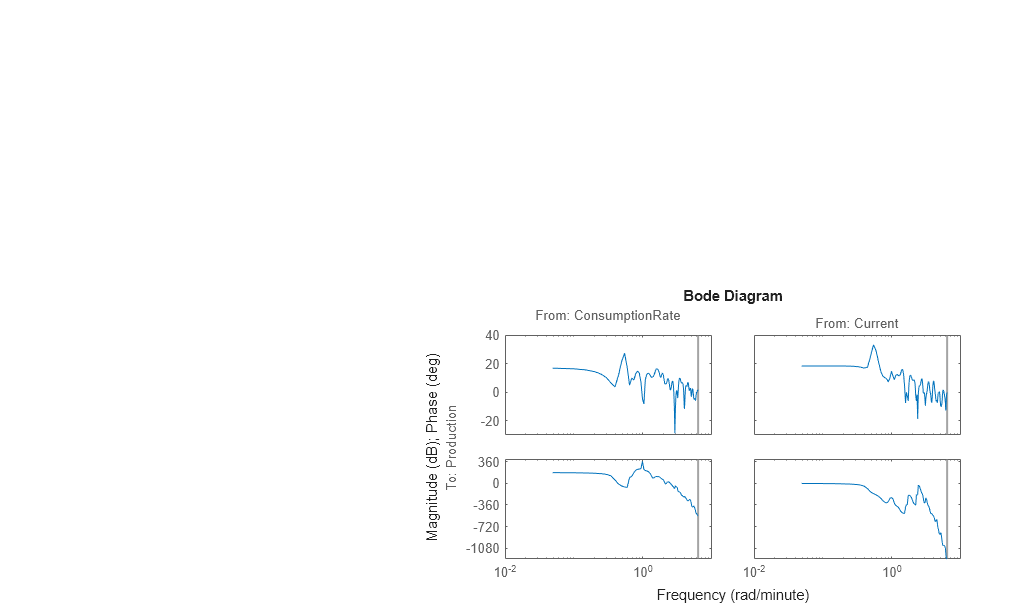The amplitude peaks at the frequency of 0.54 rad/min, which suggests a possible resonant behavior (complex poles) for the first input-to-output combination - `ConsumptionRate` to `Production`.

In both plots, the phase rolls off rapidly, which suggests a time delay for both input/output combinations.

#### Estimating the Empirical Step Response

To estimate the step response from the data, first estimate a non-parametric impulse response model (FIR filter) from data and then plot its step response.

```% model estimation Mimp = impulseest(Ze1,60); % step response step(Mimp) ```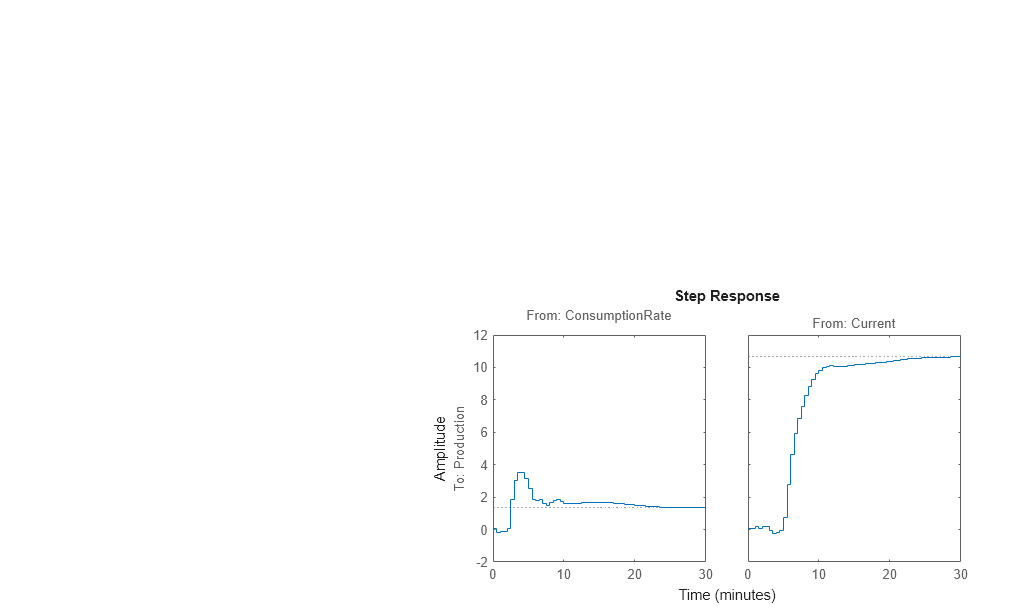The step response for the first input/output combination suggests an overshoot, which indicates the presence of an underdamped mode (complex poles) in the physical system.

The step response from the second input to the output shows no overshoot, which indicates either a first-order response or a higher-order response with real poles (overdamped response).

The step-response plot indicates a nonzero delay in the system, which is consistent with the rapid phase roll-off you got in the Bode plot you created in Estimating the Empirical Step Response.

### Estimating Delays in the Multiple-Input System

#### Why Estimate Delays?

To identify parametric black-box models, you must specify the input/output delay as part of the model order.

If you do not know the input/output delays for your system from the experiment, you can use the System Identification Toolbox software to estimate the delay.

#### Estimating Delays Using the ARX Model Structure

In the case of single-input systems, you can read the delay on the impulse-response plot. However, in the case of multiple-input systems, such as the one in this tutorial, you might be unable to tell which input caused the initial change in the output and you can use the `delayest` command instead.

The command estimates the time delay in a dynamic system by estimating a low-order, discrete-time ARX model with a range of delays, and then choosing the delay that corresponding to the best fit.

The ARX model structure is one of the simplest black-box parametric structures. In discrete-time, the ARX structure is a difference equation with the following form:

y(t) represents the output at time t, u(t) represents the input at time t, na is the number of poles, nb is the number of b parameters (equal to the number of zeros plus 1), nk is the number of samples before the input affects output of the system (called the delay or dead time of the model), and e(t) is the white-noise disturbance.

`delayest` assumes that na=nb=`2` and that the noise e is white or insignificant, and estimates nk.

To estimate the delay in this system, type:

```delayest(ze) ```
```ans = 5 10 ```

This result includes two numbers because there are two inputs: the estimated delay for the first input is `5` data samples, and the estimated delay for the second input is `10` data samples. Because the sample time for the experiments is `0.5` min, this corresponds to a `2.5` -min delay before the first input affects the output, and a `5.0` -min delay before the second input affects the output.

#### Estimating Delays Using Alternative Methods

There are two alternative methods for estimating the time delay in the system:

• Plot the time plot of the input and output data and read the time difference between the first change in the input and the first change in the output. This method is practical only for single-input/single-output system; in the case of multiple-input systems, you might be unable to tell which input caused the initial change in the output.

• Plot the impulse response of the data with a 1-standard-deviation confidence region. You can estimate the time delay using the time when the impulse response is first outside the confidence region.

### Estimating Model Orders Using an ARX Model Structure

#### Why Estimate Model Order?

Model order is one or more integers that define the complexity of the model. In general, model order is related to the number of poles, the number of zeros, and the response delay (time in terms of the number of samples before the output responds to the input). The specific meaning of model order depends on the model structure.

To compute parametric black-box models, you must provide the model order as an input. If you do not know the order of your system, you can estimate it.

After completing the steps in this section, you get the following results:

• For the first input/output combination: na=2, nb=2, and the delay nk=5.

• For the second input/output combination: na=1, nb=1, and the delay nk=10.

Later, you explore different model structures by specifying model-order values that are slight variations around these initial estimate.

#### Commands for Estimating the Model Order

In this portion of the tutorial, you use `struc`, `arxstruc`, and `selstruc` to estimate and compare simple polynomial (ARX) models for a range of model orders and delays, and select the best orders based on the quality of the model.

The following list describes the results of using each command:

• `struc` creates a matrix of model-order combinations for a specified range of na, nb, and nk values.

• `arxstruc` takes the output from `struc`, systematically estimates an ARX model for each model order, and compares the model output to the measured output. `arxstruc` returns the loss function for each model, which is the normalized sum of squared prediction errors.

• `selstruc` takes the output from `arxstruc` and opens the ARX Model Structure Selection window, which resembles the following figure, to help you choose the model order.

You use this plot to select the best-fit model.• The horizontal axis is the total number of parameters — na + nb.

• The vertical axis, called Unexplained output variance (in %), is the portion of the output not explained by the model—the ARX model prediction error for the number of parameters shown on the horizontal axis.

The prediction error is the sum of the squares of the differences between the validation data output and the model one-step-ahead predicted output.

• nk is the delay.

Three rectangles are highlighted on the plot in green, blue, and red. Each color indicates a type of best-fit criterion, as follows:

• Red — Best fit minimizes the sum of the squares of the difference between the validation data output and the model output. This rectangle indicates the overall best fit.

• Green — Best fit minimizes Rissanen MDL criterion.

• Blue — Best fit minimizes Akaike AIC criterion.

In this tutorial, the Unexplained output variance (in %) value remains approximately constant for the combined number of parameters from 4 to 20. Such constancy indicates that model performance does not improve at higher orders. Thus, low-order models might fit the data equally well.

Note

When you use the same data set for estimation and validation, use the MDL and AIC criteria to select model orders. These criteria compensate for overfitting that results from using too many parameters. For more information about these criteria, see the `selstruc` reference page.

#### Model Order for the First Input-Output Combination

In this tutorial, there are two inputs to the system and one output and you estimate model orders for each input/output combination independently. You can either estimate the delays from the two inputs simultaneously or one input at a time.

It makes sense to try the following order combinations for the first input/output combination:

• na=`2:5`

• nb=`1:5`

• nk=`5`

This is because the nonparametric models you estimated in Estimating Impulse Response Models show that the response for the first input/output combination might have a second-order response. Similarly, in Estimating Delays in the Multiple-Input System, the delay for this input/output combination was estimated to be 5.

To estimate model order for the first input/output combination:

1. Use `struc` to create a matrix of possible model orders.

`NN1 = struc(2:5,1:5,5);`
2. Use `selstruc` to compute the loss functions for the ARX models with the orders in `NN1`.

`selstruc(arxstruc(ze(:,:,1),zv(:,:,1),NN1))`

Note

`ze(:,:,1)` selects the first input in the data.

This command opens the interactive ARX Model Structure Selection window.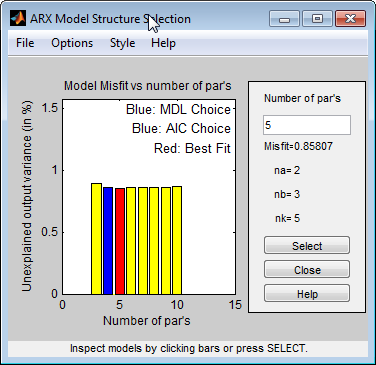Note

The Rissanen MDL and Akaike AIC criteria produces equivalent results and are both indicated by a blue rectangle on the plot.

The red rectangle represents the best overall fit, which occurs for na=2, nb=3, and nk=5. The height difference between the red and blue rectangles is insignificant. Therefore, you can choose the parameter combination that corresponds to the lowest model order and the simplest model.

3. Click the blue rectangle, and then click to choose that combination of orders:

na=`2`

nb=`2`

nk=`5`

4. To continue, press any key while in the MATLAB Command Window.

#### Model Order for the Second Input-Output Combination

It makes sense to try the following order combinations for the second input/output combination:

• na=`1:3`

• nb=`1:3`

• nk=`10`

This is because the nonparametric models you estimated in Estimating Impulse Response Models show that the response for the second input/output combination might have a first-order response. Similarly, in Estimating Delays in the Multiple-Input System, the delay for this input/output combination was estimated to be 10.

To estimate model order for the second input/output combination:

1. Use `struc` to create a matrix of possible model orders.

`NN2 = struc(1:3,1:3,10);`
2. Use `selstruc` to compute the loss functions for the ARX models with the orders in `NN2`.

`selstruc(arxstruc(ze(:,:,2),zv(:,:,2),NN2))`

This command opens the interactive ARX Model Structure Selection window.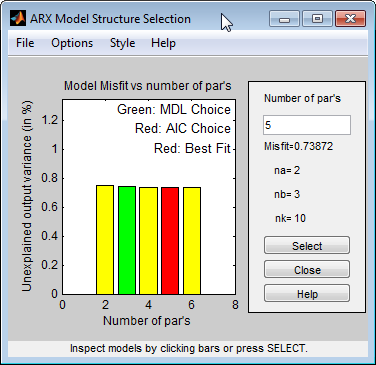Note

The Akaike AIC and the overall best fit criteria produces equivalent results. Both are indicated by the same red rectangle on the plot.

The height difference between all of the rectangles is insignificant and all of these model orders result in similar model performance. Therefore, you can choose the parameter combination that corresponds to the lowest model order and the simplest model.

3. Click the yellow rectangle on the far left, and then click to choose the lowest order: na=1, nb=1, and nk=10.

4. To continue, press any key while in the MATLAB Command Window.

### Estimating Transfer Functions

#### Specifying the Structure of the Transfer Function

In this portion of the tutorial, you estimate a continuous-time transfer function. You must have already prepared your data, as described in Preparing Data. You can use the following results of estimated model orders to specify the orders of the model:

• For the first input/output combination, use:

• Two poles, corresponding to `na=2` in the ARX model.

• Delay of 5, corresponding to `nk=5` samples (or 2.5 minutes) in the ARX model.

• For the second input/output combination, use:

• One pole, corresponding to `na=1` in the ARX model

• Delay of 10, corresponding to `nk=10` samples (or 5 minutes) in the ARX model.

You can estimate a transfer function of these orders using the `tfest` command. For the estimation, you can also choose to view a progress report by setting the `Display` option to `on` in the option set created by the `tfestOptions` command.

```Opt = tfestOptions('Display','on'); ```

Collect the model orders and delays into variables to pass to `tfest`.

```np = [2 1]; ioDelay = [2.5 5]; ```

Estimate the transfer function.

```mtf = tfest(Ze1,np,[],ioDelay,Opt); ```View the model's coefficients.

```mtf ```
```mtf = From input "ConsumptionRate" to output "Production": -1.382 s + 0.0008305 exp(-2.5*s) * ------------------------- s^2 + 1.014 s + 5.443e-12 From input "Current" to output "Production": 5.93 exp(-5*s) * ---------- s + 0.5858 Continuous-time identified transfer function. Parameterization: Number of poles: [2 1] Number of zeros: [1 0] Number of free coefficients: 6 Use "tfdata", "getpvec", "getcov" for parameters and their uncertainties. Status: Estimated using TFEST on time domain data "Ze1". Fit to estimation data: 78.92% FPE: 14.22, MSE: 13.97 ```

The model's display shows more than 85% fit to estimation data.

#### Validating the Model

In this portion of the tutorial, you create a plot that compares the actual output and the model output using the `compare` command.

```compare(Zv1,mtf) ```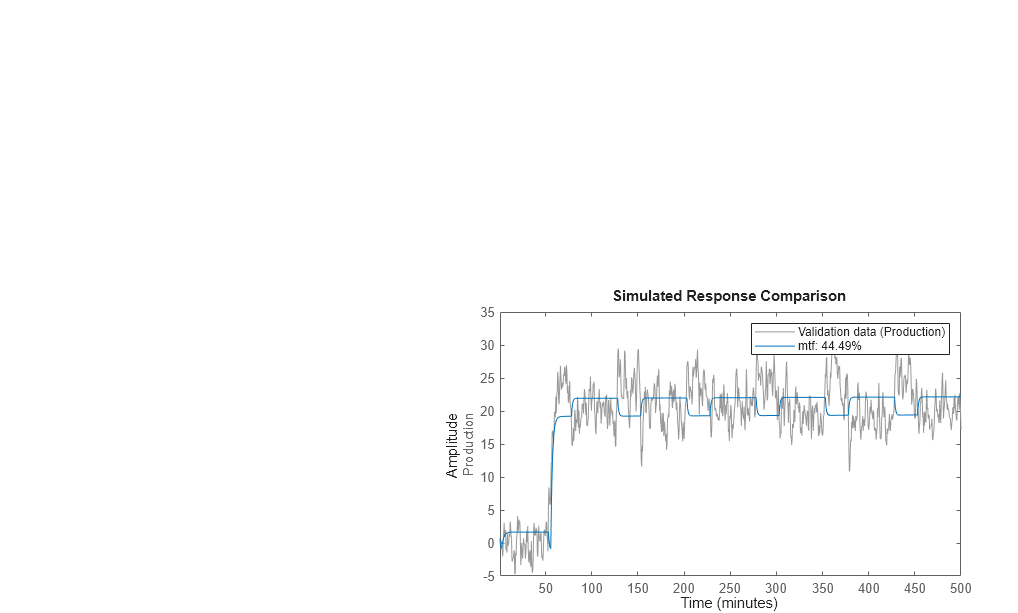The comparison shows about 60% fit.

#### Residual Analysis

Use the `resid` command to evaluate the characteristics of the residuals.

```resid(Zv1,mtf) ```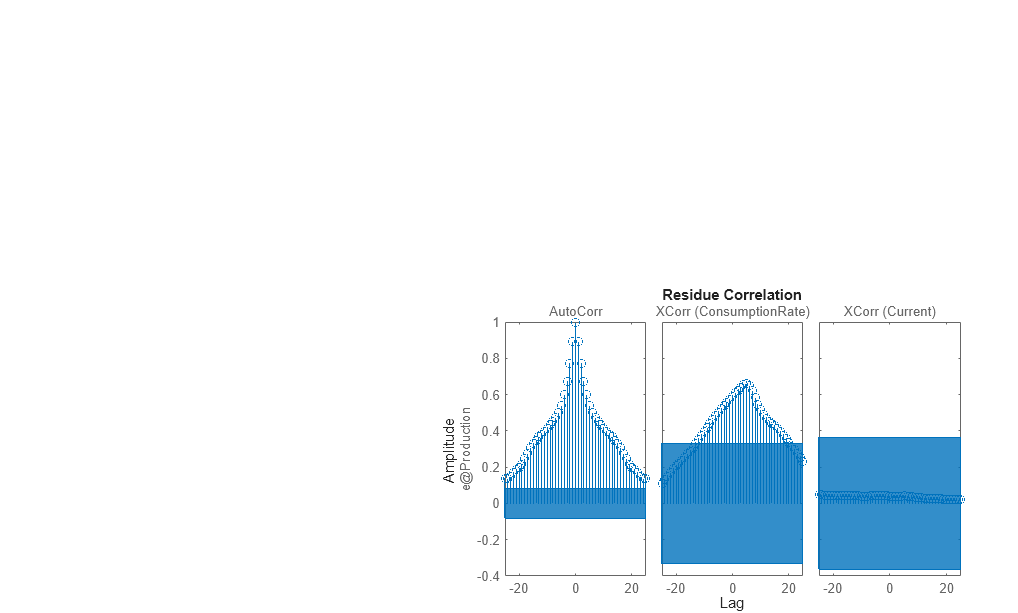The residuals show high degree of auto-correlation. This is not unexpected since the model `mtf` does not have any components to describe the nature of the noise separately. To model both the measured input-output dynamics and the disturbance dynamics you will need to use a model structure that contains elements to describe the noise component. You can use `bj`, `ssest` and `procest` commands, which create models of polynomial, state-space and process structures respectively. These structures, among others, contain elements to capture the noise behavior.

### Estimating Process Models

#### Specifying the Structure of the Process Model

In this portion of the tutorial, you estimate a low-order, continuous-time transfer function (process model). the System Identification Toolbox product supports continuous-time models with at most three poles (which might contain underdamped poles), one zero, a delay element, and an integrator.

You must have already prepared your data, as described in Preparing Data.

You can use the following results of estimated model orders to specify the orders of the model:

• For the first input/output combination, use:

• Two poles, corresponding to na=2 in the ARX model.

• Delay of 5, corresponding to nk=5 samples (or 2.5 minutes) in the ARX model.

• For the second input/output combination, use:

• One pole, corresponding to na=1 in the ARX model.

• Delay of 10, corresponding to nk=10 samples (or 5 minutes) in the ARX model.

Note

Because there is no relationship between the number of zeros estimated by the discrete-time ARX model and its continuous-time counterpart, you do not have an estimate for the number of zeros. In this tutorial, you can specify one zero for the first input/output combination, and no zero for the second-output combination.

Use the `idproc` command to create two model structures, one for each input/output combination:

```midproc0 = idproc({'P2ZUD','P1D'}, 'TimeUnit', 'minutes'); ```

The cell array `{'P2ZUD','P1D'}` specifies the model structure for each input/output combination:

• `'P2ZUD'` represents a transfer function with two poles ( `P2` ), one zero ( `Z` ), underdamped (complex-conjugate) poles ( `U` ) and a delay ( `D` ).

• `'P1D'` represents a transfer function with one pole ( `P1` ) and a delay ( `D` ).

The example also uses the `TimeUnit` parameter to specify the unit of time used.

#### Viewing the Model Structure and Parameter Values

View the two resulting models.

```midproc0 ```
```midproc0 = Process model with 2 inputs: y = G11(s)u1 + G12(s)u2 From input 1 to output 1: 1+Tz*s G11(s) = Kp * ---------------------- * exp(-Td*s) 1+2*Zeta*Tw*s+(Tw*s)^2 Kp = NaN Tw = NaN Zeta = NaN Td = NaN Tz = NaN From input 2 to output 1: Kp G12(s) = ---------- * exp(-Td*s) 1+Tp1*s Kp = NaN Tp1 = NaN Td = NaN Parameterization: {'P2DUZ'} {'P1D'} Number of free coefficients: 8 Use "getpvec", "getcov" for parameters and their uncertainties. Status: Created by direct construction or transformation. Not estimated. ```

The parameter values are set to `NaN` because they are not yet estimated.

#### Specifying Initial Guesses for Time Delays

Set the time delay property of the model object to `2.5` min and `5` min for each input/output pair as initial guesses. Also, set an upper limit on the delay because good initial guesses are available.

```midproc0.Structure(1,1).Td.Value = 2.5; midproc0.Structure(1,2).Td.Value = 5; midproc0.Structure(1,1).Td.Maximum = 3; midproc0.Structure(1,2).Td.Maximum = 7; ```

Note

When setting the delay constraints, you must specify the delays in terms of actual time units (minutes, in this case) and not the number of samples.

#### Estimating Model Parameters Using procest

`procest` is an iterative estimator of process models, which means that it uses an iterative nonlinear least-squares algorithm to minimize a cost function. The cost function is the weighted sum of the squares of the errors.

Depending on its arguments, `procest` estimates different black-box polynomial models. You can use `procest`, for example, to estimate parameters for linear continuous-time transfer-function, state-space, or polynomial model structures. To use `procest`, you must provide a model structure with unknown parameters and the estimation data as input arguments.

For this portion of the tutorial, you must have already defined the model structure, as described in Specifying the Structure of the Process Model. Use `midproc0` as the model structure and `Ze1` as the estimation data:

```midproc = procest(Ze1,midproc0); present(midproc) ```
``` midproc = Process model with 2 inputs: y = G11(s)u1 + G12(s)u2 From input "ConsumptionRate" to output "Production": 1+Tz*s G11(s) = Kp * ---------------------- * exp(-Td*s) 1+2*Zeta*Tw*s+(Tw*s)^2 Kp = -1.1807 +/- 0.29986 Tw = 1.6437 +/- 714.6 Zeta = 16.036 +/- 6958.9 Td = 2.426 +/- 64.276 Tz = -109.19 +/- 63.735 From input "Current" to output "Production": Kp G12(s) = ---------- * exp(-Td*s) 1+Tp1*s Kp = 10.264 +/- 0.048404 Tp1 = 2.049 +/- 0.054901 Td = 4.9175 +/- 0.034374 Parameterization: {'P2DUZ'} {'P1D'} Number of free coefficients: 8 Use "getpvec", "getcov" for parameters and their uncertainties. Status: Termination condition: Maximum number of iterations reached.. Number of iterations: 20, Number of function evaluations: 279 Estimated using PROCEST on time domain data "Ze1". Fit to estimation data: 86.2% FPE: 6.081, MSE: 5.984 More information in model's "Report" property. ```

Unlike discrete-time polynomial models, continuous-time models let you estimate the delays. In this case, the estimated delay values are different from the initial guesses you specified of `2.5` and `5`, respectively. The large uncertainties in the estimated values of the parameters of `G_1(s)` indicate that the dynamics from input `1` to the output are not captured well.

#### Validating the Model

In this portion of the tutorial, you create a plot that compares the actual output and the model output.

```compare(Zv1,midproc) ```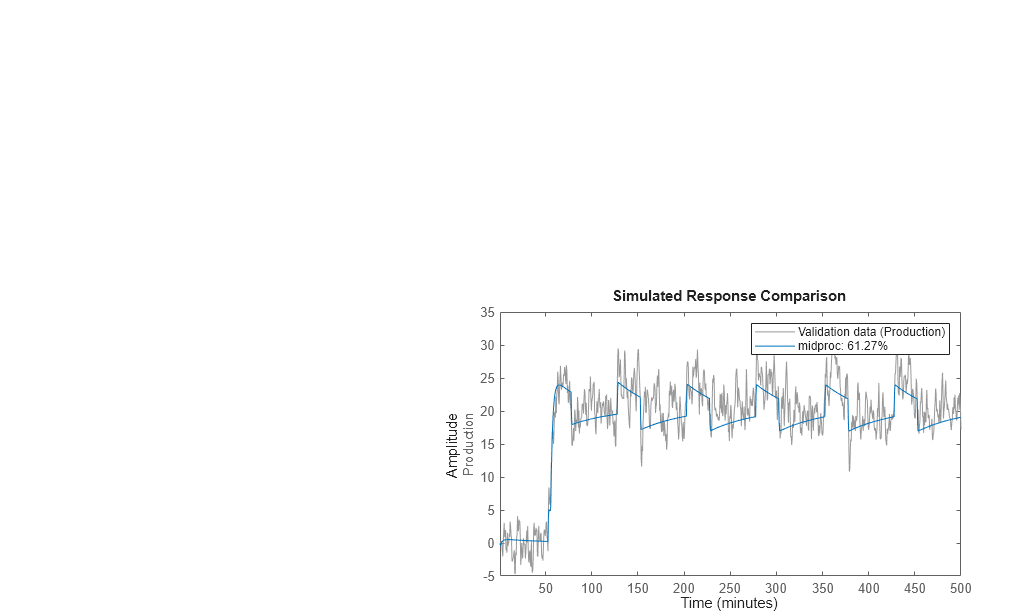The plot shows that the model output reasonably agrees with the measured output: there is an agreement of 60% between the model and the validation data.

Use `resid` to perform residual analysis.

```resid(Zv1,midproc) ```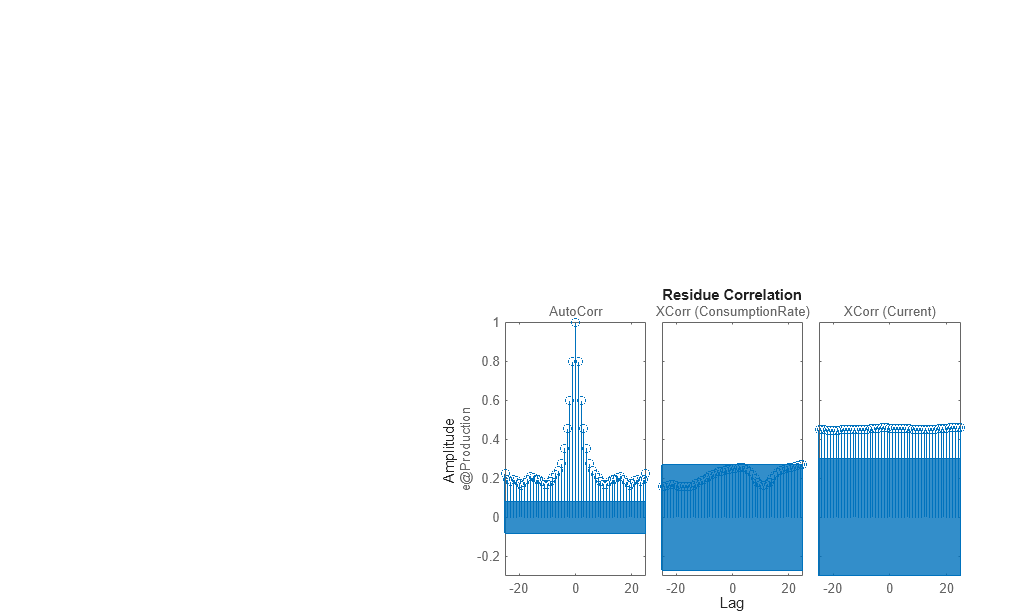The cross-correlation between the second input and residual errors is significant. The autocorrelation plot shows values outside the confidence region and indicates that the residuals are correlated.

Change the algorithm for iterative parameter estimation to Levenberg-Marquardt.

```Opt = procestOptions; Opt.SearchMethod = 'lm'; midproc1 = procest(Ze1,midproc,Opt); ```

Tweaking the algorithm properties or specifying initial parameter guesses and rerunning the estimation may improve the simulation results. Adding a noise model may improve prediction results but not necessarily the simulation results.

#### Estimating a Process Model with Noise Model

This portion of the tutorial shows how to estimate a process model and include a noise model in the estimation. Including a noise model typically improves model prediction results but not necessarily the simulation results.

Use the following commands to specify a first-order ARMA noise:

```Opt = procestOptions; Opt.DisturbanceModel = 'ARMA1'; midproc2 = procest(Ze1,midproc0,Opt); ```

You can type `'dist'` instead of `'DisturbanceModel'`. Property names are not case sensitive, and you only need to include the portion of the name that uniquely identifies the property.

Compare the resulting model to the measured data.

```compare(Zv1,midproc2) ```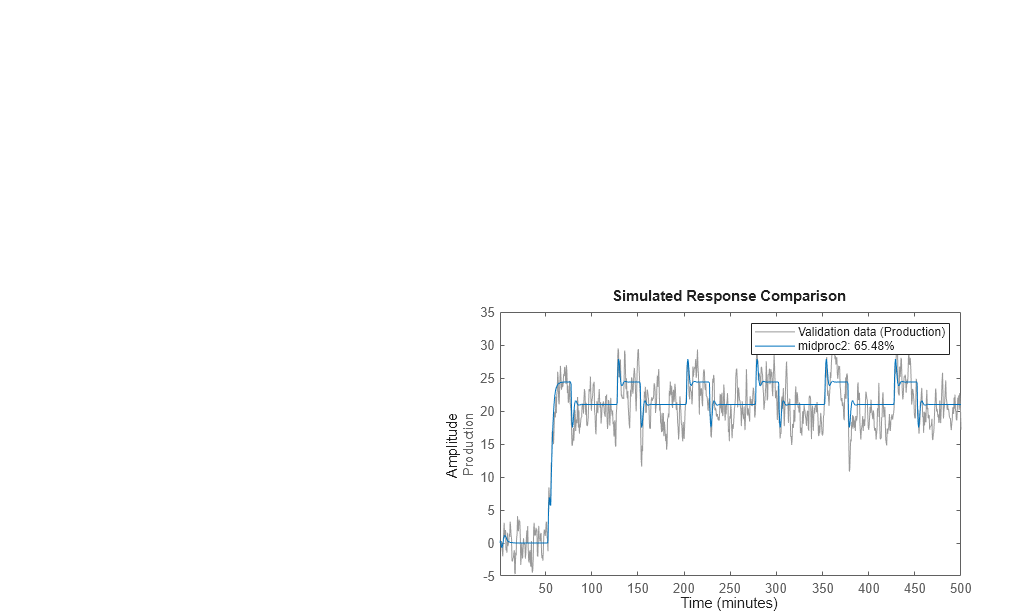The plot shows that the model output maintains reasonable agreement with the validation-data output.

Perform residual analysis.

```resid(Zv1,midproc2) ```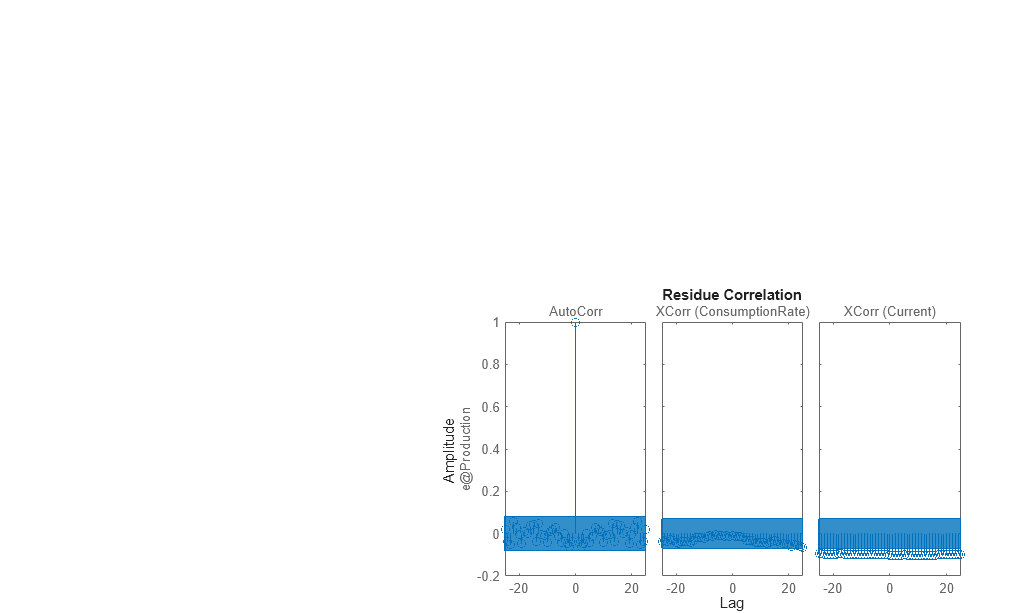The residual plot shows that autocorrelation values are inside the confidence bounds. Thus adding a noise model produces uncorrelated residuals. This indicates a more accurate model.

### Estimating Black-Box Polynomial Models

#### Model Orders for Estimating Polynomial Models

In this portion of the tutorial, you estimate several different types of black-box, input-output polynomial models.

You must have already prepared your data, as described in Preparing Data.

You can use the following previous results of estimated model orders to specify the orders of the polynomial model:

• For the first input/output combination, use:

• Two poles, corresponding to na=2 in the ARX model.

• One zero, corresponding to nb=2 in the ARX model.

• Delay of 5, corresponding to nk=5 samples (or 2.5 minutes) in the ARX model.

• For the second input/output combination, use:

• One pole, corresponding to na=1 in the ARX model.

• No zeros, corresponding to nb=1 in the ARX model.

• Delay of 10, corresponding to nk=10 samples (or 5 minutes) in the ARX model.

#### Estimating a Linear ARX Model

About ARX Models.  For a single-input/single-output system (SISO), the ARX model structure is:

y(t) represents the output at time t, u(t) represents the input at time t, na is the number of poles, nb is the number of zeros plus 1, nk is the number of samples before the input affects output of the system (called the delay or dead time of the model), and e(t) is the white-noise disturbance.

The ARX model structure does not distinguish between the poles for individual input/output paths: dividing the ARX equation by A, which contains the poles, shows that A appears in the denominator for both inputs. Therefore, you can set na to the sum of the poles for each input/output pair, which is equal to `3` in this case.

The System Identification Toolbox product estimates the parameters ${a}_{1}\dots {a}_{n}$ and ${b}_{1}\dots {b}_{n}$ using the data and the model orders you specify.

Estimating ARX Models Using arx.  Use `arx` to compute the polynomial coefficients using the fast, noniterative method `arx`:

```marx = arx(Ze1,'na',3,'nb',[2 1],'nk',[5 10]); present(marx) % Displays model parameters % with uncertainty information ```
``` marx = Discrete-time ARX model: A(z)y(t) = B(z)u(t) + e(t) A(z) = 1 - 1.027 (+/- 0.02907) z^-1 + 0.1678 (+/- 0.042) z^-2 + 0.01289 ( +/- 0.02583) z^-3 B1(z) = 1.86 (+/- 0.189) z^-5 - 1.608 (+/- 0.1888) z^-6 B2(z) = 1.612 (+/- 0.07392) z^-10 Sample time: 0.5 minutes Parameterization: Polynomial orders: na=3 nb=[2 1] nk=[5 10] Number of free coefficients: 6 Use "polydata", "getpvec", "getcov" for parameters and their uncertainties. Status: Estimated using ARX on time domain data "Ze1". Fit to estimation data: 90.7% (prediction focus) FPE: 2.768, MSE: 2.719 More information in model's "Report" property. ```

MATLAB estimates the polynomials `A` , `B1` , and `B2`. The uncertainty for each of the model parameters is computed to 1 standard deviation and appears in parentheses next to each parameter value.

Alternatively, you can use the following shorthand syntax and specify model orders as a single vector:

```marx = arx(Ze1,[3 2 1 5 10]); ```

Accessing Model Data.  The model you estimated, `marx`, is a discrete-time `idpoly` object. To get the properties of this model object, you can use the `get` function:

```get(marx) ```
``` A: [1 -1.0267 0.1678 0.0129] B: {[0 0 0 0 0 1.8599 -1.6084] [0 0 0 0 0 0 0 0 0 0 1.6118]} C: 1 D: 1 F: { } IntegrateNoise: 0 Variable: 'z^-1' IODelay: [0 0] Structure: [1x1 pmodel.polynomial] NoiseVariance: 2.7436 InputDelay: [2x1 double] OutputDelay: 0 InputName: {2x1 cell} InputUnit: {2x1 cell} InputGroup: [1x1 struct] OutputName: {'Production'} OutputUnit: {'mg/min'} OutputGroup: [1x1 struct] Notes: [0x1 string] UserData: [] Name: '' Ts: 0.5000 TimeUnit: 'minutes' SamplingGrid: [1x1 struct] Report: [1x1 idresults.arx] ```

You can access the information stored by these properties using dot notation. For example, you can compute the discrete poles of the model by finding the roots of the `A` polynomial.

```marx_poles = roots(marx.a) ```
```marx_poles = 0.7953 0.2877 -0.0564 ```

In this case, you access the `A` polynomial using `marx.a`.

The model `marx` describes system dynamics using three discrete poles.

Tip

You can also use `pole` to compute the poles of a model directly.

#### Estimating State-Space Models

About State-Space Models.  The general state-space model structure is:

`$\begin{array}{l}\frac{dx}{dt}=Ax\left(t\right)+Bu\left(t\right)+Ke\left(t\right)\\ y\left(t\right)=Cx\left(t\right)+Du\left(t\right)+e\left(t\right)\end{array}$`

y(t) represents the output at time t, u(t) represents the input at time t, x(t) is the state vector at time t, and e(t) is the white-noise disturbance.

You must specify a single integer as the model order (dimension of the state vector) to estimate a state-space model. By default, the delay equals `1`.

The System Identification Toolbox product estimates the state-space matrices A, B, C, D, and K using the model order and the data you specify.

The state-space model structure is a good choice for quick estimation because it contains only two parameters: `n` is the number of poles (the size of the A matrix) and `nk` is the delay.

Estimating State-Space Models Using n4sid.  Use the `n4sid` command to specify a range of model orders and evaluate the performance of several state-space models (orders 2 to 8):

`mn4sid = n4sid(Ze1,2:8,'InputDelay',[4 9]);`

This command uses the fast, noniterative (subspace) method and opens the following plot. You use this plot to decide which states provide a significant relative contribution to the input/output behavior, and which states provide the smallest contribution.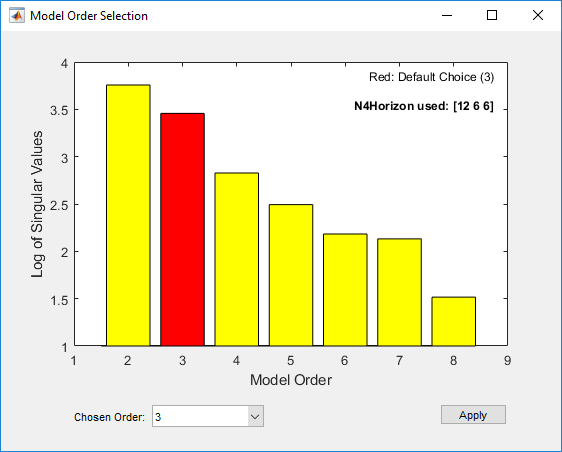The vertical axis is a relative measure of how much each state contributes to the input/output behavior of the model (log of singular values of the covariance matrix). The horizontal axis corresponds to the model order `n`. This plot recommends `n=3`, indicated by a red rectangle.

The Chosen Order order field displays the recommended model order, `3` in this case, by default. You can change the order selection by using the Chosen Order drop-down list. Apply the value in the Chosen Order field and close the order-selection window by clicking .

By default, `n4sid` uses a free parameterization of the state-space form. To estimate a canonical form instead, set the value of the `SSParameterization` property to `'Canonical'`. You can also specify the input-to-output delay (in samples) using the `'InputDelay'` property.

```mCanonical = n4sid(Ze1,3,'SSParameterization','canonical','InputDelay',[4 9]); present(mCanonical); % Display model properties ```
``` mCanonical = Discrete-time identified state-space model: x(t+Ts) = A x(t) + B u(t) + K e(t) y(t) = C x(t) + D u(t) + e(t) A = x1 x2 x3 x1 0 1 0 x2 0 0 1 x3 0.0737 +/- 0.05919 -0.6093 +/- 0.1626 1.446 +/- 0.1287 B = ConsumptionR Current x1 1.844 +/- 0.175 0.5633 +/- 0.122 x2 1.063 +/- 0.1673 2.308 +/- 0.1222 x3 0.2779 +/- 0.09615 1.878 +/- 0.1058 C = x1 x2 x3 Production 1 0 0 D = ConsumptionR Current Production 0 0 K = Production x1 0.8674 +/- 0.03169 x2 0.6849 +/- 0.04145 x3 0.5105 +/- 0.04352 Input delays (sampling periods): 4 9 Sample time: 0.5 minutes Parameterization: CANONICAL form with indices: 3. Feedthrough: none Disturbance component: estimate Number of free coefficients: 12 Use "idssdata", "getpvec", "getcov" for parameters and their uncertainties. Status: Estimated using N4SID on time domain data "Ze1". Fit to estimation data: 91.39% (prediction focus) FPE: 2.402, MSE: 2.331 More information in model's "Report" property. ```

Note

`mn4sid` and `mCanonical` are discrete-time models. To estimate a continuous-time model, set the `'Ts'` property to `0` in the estimation command, or use the `ssest` command:

```mCT1 = n4sid(Ze1, 3, 'Ts', 0, 'InputDelay', [2.5 5]) mCT2 = ssest(Ze1, 3,'InputDelay', [2.5 5]) ```

#### Estimating a Box-Jenkins Model

About Box-Jenkins Models.  The general Box-Jenkins (BJ) structure is:

`$y\left(t\right)=\sum _{i=1}^{nu}\frac{{B}_{i}\left(q\right)}{{F}_{i}\left(q\right)}{u}_{i}\left(t-n{k}_{i}\right)+\frac{C\left(q\right)}{D\left(q\right)}e\left(t\right)$`

To estimate a BJ model, you need to specify the parameters nb, nf, nc, nd, and nk.

Whereas the ARX model structure does not distinguish between the poles for individual input/output paths, the BJ model provides more flexibility in modeling the poles and zeros of the disturbance separately from the poles and zeros of the system dynamics.

Estimating a BJ Model Using polyest.  You can use `polyest` to estimate the BJ model. `polyest` is an iterative method and has the following general syntax:

`polyest(data,[na nb nc nd nf nk]);`

To estimate the BJ model, type:

```na = 0; nb = [ 2 1 ]; nc = 1; nd = 1; nf = [ 2 1 ]; nk = [ 5 10]; mbj = polyest(Ze1,[na nb nc nd nf nk]); ```

This command specifies `nf=2` , `nb=2` , `nk=5` for the first input, and `nf=nb=1` and `nk=10` for the second input.

Display the model information.

```present(mbj) ```
``` mbj = Discrete-time BJ model: y(t) = [B(z)/F(z)]u(t) + [C(z)/D(z)]e(t) B1(z) = 1.823 (+/- 0.1792) z^-5 - 1.315 (+/- 0.2367) z^-6 B2(z) = 1.791 (+/- 0.06431) z^-10 C(z) = 1 + 0.1068 (+/- 0.04009) z^-1 D(z) = 1 - 0.7452 (+/- 0.02694) z^-1 F1(z) = 1 - 1.321 (+/- 0.06936) z^-1 + 0.5911 (+/- 0.05514) z^-2 F2(z) = 1 - 0.8314 (+/- 0.006441) z^-1 Sample time: 0.5 minutes Parameterization: Polynomial orders: nb=[2 1] nc=1 nd=1 nf=[2 1] nk=[5 10] Number of free coefficients: 8 Use "polydata", "getpvec", "getcov" for parameters and their uncertainties. Status: Termination condition: Near (local) minimum, (norm(g) < tol).. Number of iterations: 6, Number of function evaluations: 13 Estimated using POLYEST on time domain data "Ze1". Fit to estimation data: 90.75% (prediction focus) FPE: 2.733, MSE: 2.689 More information in model's "Report" property. ```

The uncertainty for each of the model parameters is computed to 1 standard deviation and appears in parentheses next to each parameter value.

The polynomials `C` and `D` give the numerator and the denominator of the noise model, respectively.

Tip

Alternatively, you can use the following shorthand syntax that specifies the orders as a single vector:

`mbj = bj(Ze1,[2 1 1 1 2 1 5 10]);`

`bj` is a version of `polyest` that specifically estimates the BJ model structure.

#### Comparing Model Output to Measured Output

Compare the output of the ARX, state-space, and Box-Jenkins models to the measured output.

```compare(Zv1,marx,mn4sid,mbj) ````compare` plots the measured output in the validation data set against the simulated output from the models. The input data from the validation data set serves as input to the models.

Perform residual analysis on the ARX, state-space, and Box-Jenkins models.

```resid(Zv1,marx,mn4sid,mbj) ```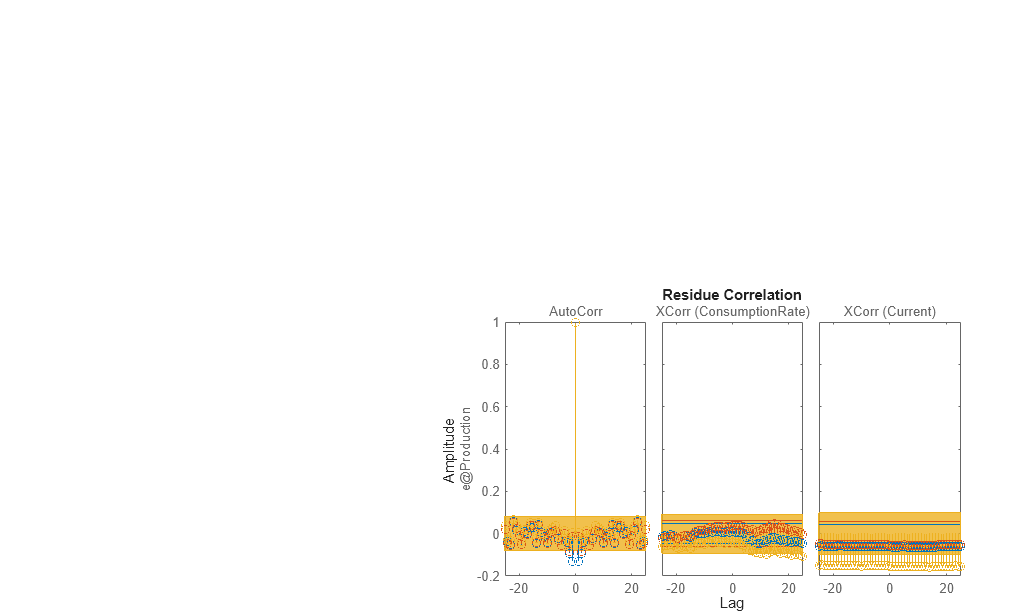All three models simulate the output equally well and have uncorrelated residuals. Therefore, choose the ARX model because it is the simplest of the three input-output polynomial models and adequately captures the process dynamics.

### Simulating and Predicting Model Output

#### Simulating the Model Output

In this portion of the tutorial, you simulate the model output. You must have already created the continuous-time model `midproc2`, as described in Estimating Process Models.

Simulating the model output requires the following information:

• Input values to the model

• Initial conditions for the simulation (also called initial states)

For example, the following commands use the `iddata` and `idinput` commands to construct an input data set, and use `sim` to simulate the model output:

```% Create input for simulation U = iddata([],idinput([200 2]),'Ts',0.5,'TimeUnit','min'); % Simulate the response setting initial conditions equal to zero ysim_1 = sim(midproc2,U); ```

To maximize the fit between the simulated response of a model to the measured output for the same input, you can compute the initial conditions corresponding to the measured data. The best fitting initial conditions can be obtained by using `findstates` on the state-space version of the estimated model. The following commands estimate the initial states `X0est` from the data set `Zv1`:

```% State-space version of the model needed for computing initial states midproc2_ss = idss(midproc2); X0est = findstates(midproc2_ss,Zv1); ```

Next, simulate the model using the initial states estimated from the data.

```% Simulation input Usim = Zv1(:,[],:); Opt = simOptions('InitialCondition',X0est); ysim_2 = sim(midproc2_ss,Usim,Opt); ```

Compare the simulated and the measured output on a plot.

```figure plot([ysim_2.y, Zv1.y]) legend({'model output','measured'}) xlabel('time'), ylabel('Output') ```#### Predicting the Future Output

Many control-design applications require you to predict the future outputs of a dynamic system using the past input/output data.

For example, use `predict` to predict the model response five steps ahead:

```predict(midproc2,Ze1,5) ```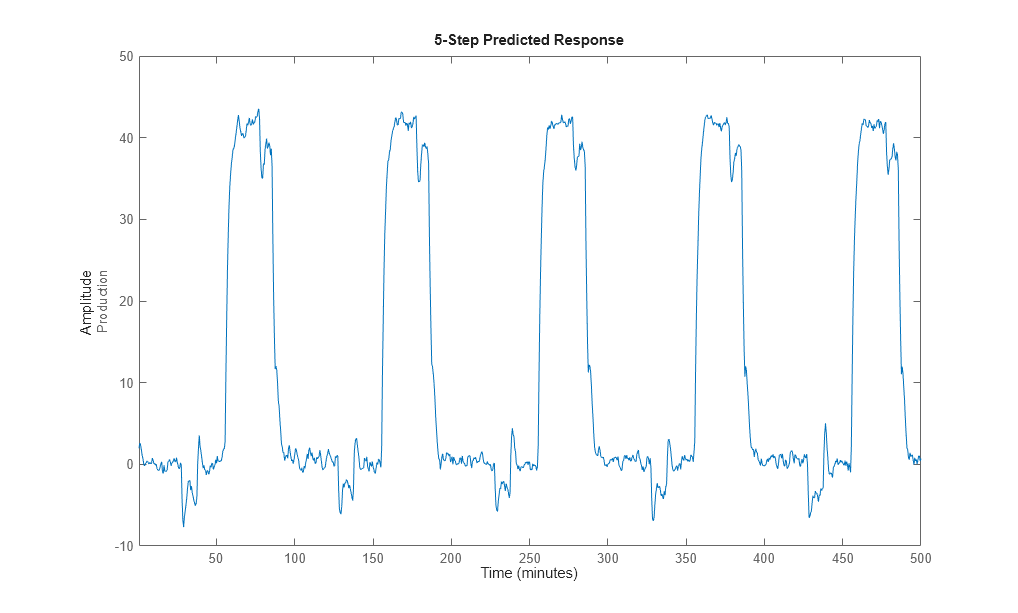Compare the predicted output values with the measured output values. The third argument of `compare` specifies a five-step-ahead prediction. When you do not specify a third argument, `compare` assumes an infinite prediction horizon and simulates the model output instead.

```compare(Ze1,midproc2,5) ```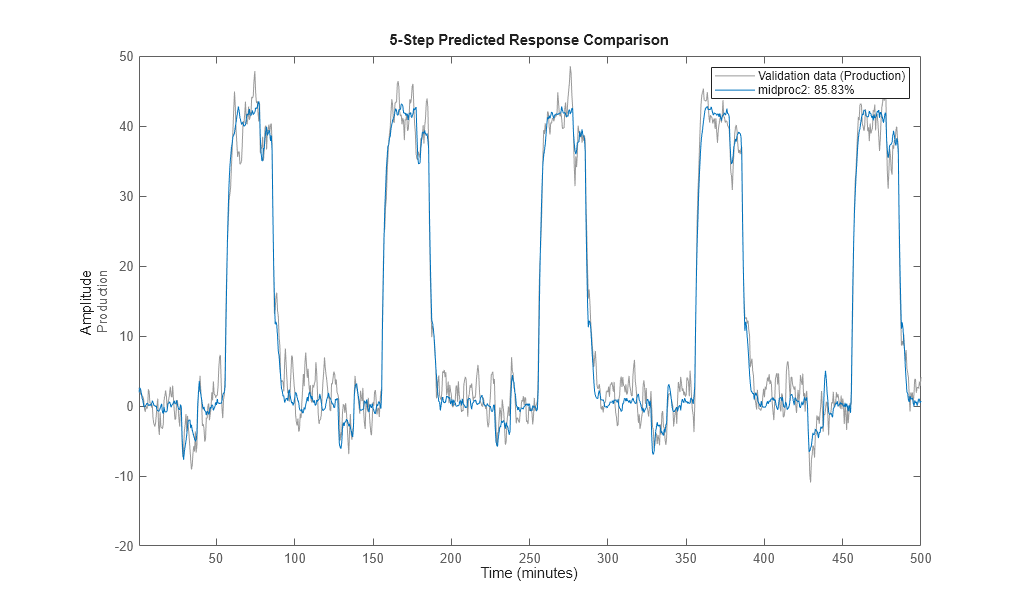Use `pe` to compute the prediction error `Err` between the predicted output of `midproc2` and the measured output. Then, plot the error spectrum using the `spectrum` command.

```[Err] = pe(midproc2,Zv1); spectrum(spa(Err,[],logspace(-2,2,200))) ```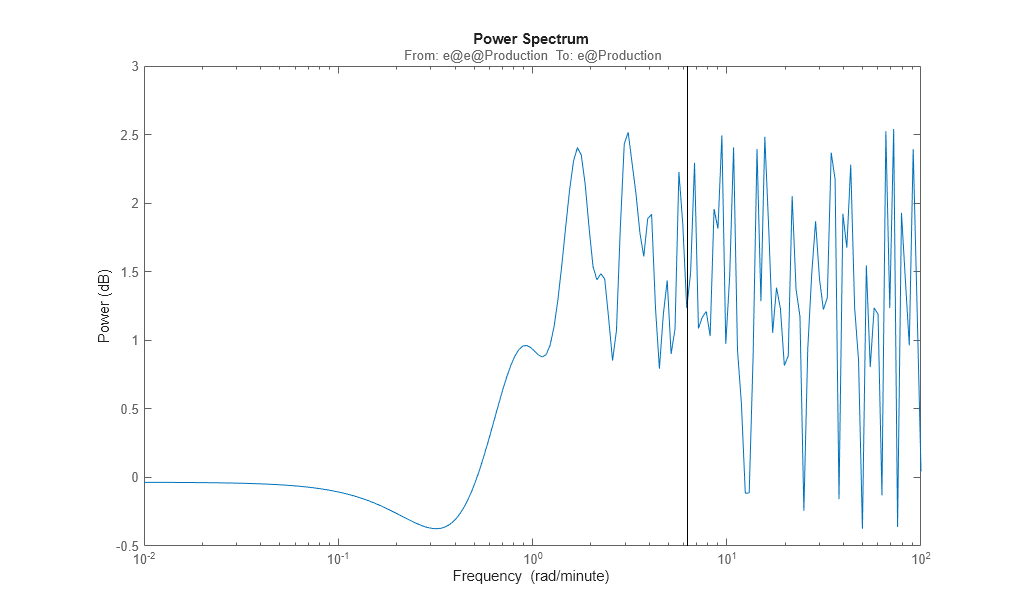The prediction errors are plotted with a 1-standard-deviation confidence interval. The errors are greater at high frequencies because of the high-frequency nature of the disturbance.